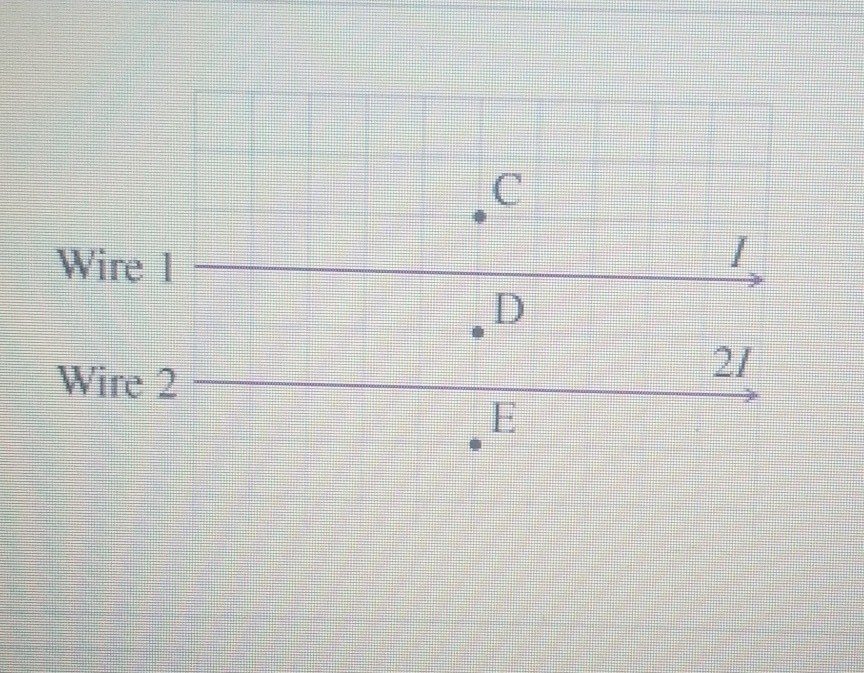# Problem: What is the direction of the magnetic field Bc at Point C?a. Bc is out of the pageb. Bc is into the pagec. Bc is neither out of nor into the page and Bc ≠ 0d. Bc = 0

###### FREE Expert Solution

The magnetic field at point C due to Wire 1 is:

$\overline{){\mathbf{B}}{\mathbf{=}}\frac{{\mathbf{\mu }}_{\mathbf{0}}}{\mathbf{2}\mathbf{\pi }}{\mathbf{·}}\frac{\mathbf{I}}{\mathbf{r}}}$, which is out of the page.

The magnetic field at point C due to Wire 2 is:

$\overline{){\mathbf{B}}{\mathbf{=}}\frac{{\mathbf{\mu }}_{\mathbf{0}}}{\mathbf{2}\mathbf{\pi }}{\mathbf{·}}\frac{\mathbf{2}\mathbf{I}}{\mathbf{3}\mathbf{r}}}$, which is also out of the page.

94% (428 ratings)###### Problem DetailsWhat is the direction of the magnetic field Bc at Point C?
a. Bc is out of the page
b. Bc is into the page
c. Bc is neither out of nor into the page and Bc ≠ 0
d. Bc = 0